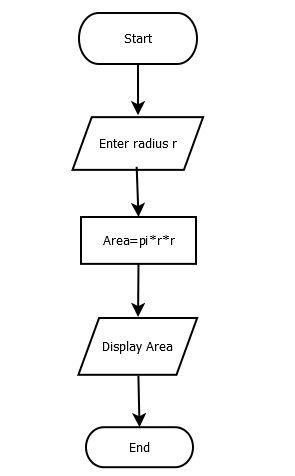# Write a Program to Find Area of Circle

Area of a circle can be found by multiplying a constant (pi) by square of the radius. You can refer Algorithm and flowchart of this program as well for better understanding.

#### Flowchart:#### Algorithm:

Begin:

Step1: Declare a constant PI=3.14

Step2: Take a numeric value (radius) from the user

Step3: Multiply square of the radius by constant (PI) and store result into a new variable

Step4: Display the result

End:

#### Program Example

This program is tested and executed using Atom IDE with Python 3.8 version. To find area, we cast user input to float because input function returns string type value.

PI = 3.14